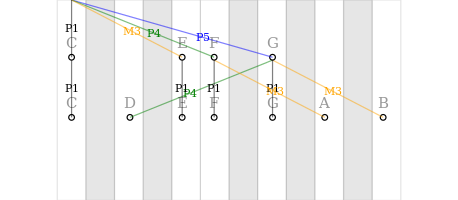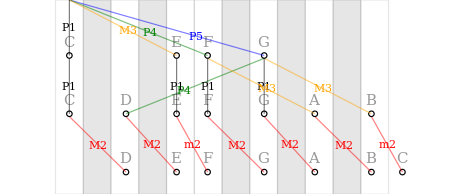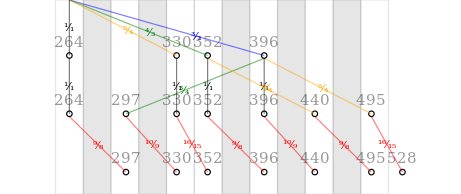In :
import Graphics.Dynamic.Plot.R2
import Data.Function
import Data.VectorSpace
import Data.Colour.Names

In :
tone :: Double -> Double
tone t = case t - fromIntegral (round t::Int) of
φ | φ>0        -> φ
| otherwise  -> φ^2

In :
chordWvFormPlots :: [Double] -> [DynamicPlottable]
chordWvFormPlots freqs = [plotLatest
[ plotMultiple (
let sigs = [ tone . (ν*) | ν <- freqs ]
amplitudes = [if t > t₀ then min 1 $t-t₀ else 0 | t₀<-[2,4..]] in [ continFnPlot ((+y₀) . (*a) . sig . (t+)) & legendName (show ν++" Hz") | ((ν, y₀), (a, sig)) <- zip (zip freqs [1..]) (zip amplitudes sigs) ] ++ [continFnPlot (sumV (zipWith ((.).(*)) amplitudes sigs) . (t+)) & tint white] ) & plotDelay 0.05 | t <- [0, 0.05 ..] ] , noDynamicAxes , forceXRange (0,4) ]  Are intervals like major 3rd, minor 3rd, and major 2nd all based on the scales, or are they based on how many semitones they have? Neither, really. They're based on frequency ratios. The consonant Western (Ptolemaic) intervals are defined as: • Octave (P8) = 2:1 • Perfect fifth (P5) = 3:2 • Perfect fourth (P4) = 4:3 • Major third (M3) = 5:4 • Major sixth (M6) = P4∗M3 = 5:3 • Minor third (m3) = P5 ⁄ M3 = 6:5 • Minor sixth (m6) = P8 ⁄ M3 = 8:5 No semitones nor scales anywhere to be seen. The reason we chose simple integer ratios: frequencies that are at a small integer ratio give nice, consistent sound signals. For example, a major chord has the following waveform: In : plotWindow$ chordWvFormPlots [4,5,6]

GraphWindowSpecR2{lBound=0.0, rBound=4.0, bBound=-0.5, tBound=3.5, xResolution=640, yResolution=480}which is much richer than the individual components, but still has a clear repetitive structure that the ear can latch onto. Compare this to a chord composed of random-ish frequencies in a similar range:

In :
plotWindow $chordWvFormPlots [3.7,5.09,6.02]  GraphWindowSpecR2{lBound=0.0, rBound=4.0, bBound=-0.5, tBound=3.5, xResolution=640, yResolution=480} which is just a noisy mess. From these fundamental intervals, the Ptolemaic scale is constructed: In : {-# LANGUAGE FlexibleContexts #-} import Diagrams.Prelude import Diagrams.Backend.Cairo (Cairo) import Control.Monad type Dia = QDiagram Cairo V2 Double Any import Data.Ratio  In : type Freq = Double -- relative to C  In : keyboard :: Dia keyboard = hcat [ rect 1 7 & fc (c k) & opacity 0.1 | k <- " ❚ ❚ ❚ ❚ ❚ " ] where c ' ' = white c '❚' = black  In : labelFreq :: Freq -> String labelFreq ν = noteNames!!s where lν = logBase 2 ν s = round$ lν * 12
noteNames = cycle $words "C C♯ D E♭ E F F♯ G G♯ A B♭ B" showRatio :: Rational -> String showRatio q = map toSup (show$ numerator q) ++ "⁄" ++ map toSub (show $denominator q) where toSup = ("⁰¹²³⁴⁵⁶⁷⁸⁹"!!) . subtract (fromEnum '0') . fromEnum toSub = ("₀₁₂₃₄₅₆₇₈₉"!!) . subtract (fromEnum '0') . fromEnum showIntervalName :: Rational -> String showIntervalName 1 = "P1" showIntervalName q | q < 1 = showIntervalName$ recip q
| q < 10/9  = "m2"
| q <= 9/8  = "M2"
| q <= 6/5  = "m3"
| q <  4/3  = "M3"
| q <  7/5  = "P4"
| q <= 3/2  = "P5"
| q <  5/3  = "m6"
| q <  7/4  = "M6"
| q < 15/8  = "m7"
| q < 2     = "M7"

In :
import Data.Monoid hiding ((<>))
import Data.Semigroup
data ScTree = ScNode
| ScBranches [(Rational, ScTree)] deriving (Show)
instance Semigroup ScTree where
ScNode<>a = a
a<>ScNode = a
ScBranches ol<>ScBranches or = ScBranches $ol++or instance Monoid ScTree where mempty = ScNode mappend = (<>) instance Num ScTree where fromInteger = fromRational . fromInteger ScNode * a = a ScBranches brs * c = ScBranches [(r, br*c) | (r,br)<-brs] instance Fractional ScTree where fromRational r = ScBranches [(r, ScNode)] ScBranches brs / ScBranches [(d,ScNode)] = ScBranches [(r/d, br)|(r,br)<-brs] :opt no-lint  In : constructScale :: (Freq -> String) -> (Rational -> String) -> ScTree -> Dia constructScale showFreq showIntv = go 1 where go _ ScNode = mempty go ν₀ (ScBranches brs) = mconcat [ (fromVertices [ p₀^&2, pn^&0 ] # opacity 0.5 # lc col <> circle 0.1 # moveTo (pn^&0) <> text (showIntv qν) # scale 0.3 # moveTo ((p₀*η+pn*(1-η))^&(2*η)) # fc col <> text (showFreq νn) # scale 0.4 # moveTo (pn^& 0.5) # opacity 0.4 ) === go νn br | (qν, br) <- brs , let νn = ν₀ * realToFrac qν [p₀,pn] = (*12) . logBase 2 <$> [ν₀,νn]
η = cos (abs . logBase 2 fromRational qν) ^ 2 / 2 iname = showIntervalName qν col = case iname of "P5" -> blue "P4" -> green (_:'2':_) -> red ('M':_) -> orange ('m':_) -> purple _ -> black ] onKeyboard :: Dia -> Dia onKeyboard pth = strutX 2 ||| (pth # alignT <> keyboard # alignT) ||| strutX 2  In : onKeyboard constructScale labelFreq showIntervalName
( 1 * 1
<> 5/4 * 1
<> 4/3 * (1 <> 5/4)
<> 3/2 * (1 <> 5/4 <> 3/4) )In this scale, there are now also other intervals apart from the consonant ones, in particular, the intervals between neighbouring scale degrees, which are called seconds.

In :
onKeyboard $constructScale labelFreq showIntervalName ( 1 * 1 * (9/8) <> 5/4 * 1 * (16/15) <> 4/3 * (1 * (9/8) <> 5/4 * (9/8)) <> 3/2 * (1 * (10/9) <> 5/4 * (16/15) <> 3/4 * (10/9)) )Here it is obvious why we talk of minor and major seconds: e.g. the distance (i.e. cross-ratio) between F and G is clearly much more than the distance between E and F, but at first glance seems to be at least similar to the distance between C and D, between D and E etc.. Here are the numerical values: In : onKeyboard$ constructScale (show . round . (440*3/5*)) showRatio
( 1 * 1 * (9/8)
<> 5/4 * 1 * (16/15)
<> 4/3 * (1 * (9/8) <> 5/4 * (9/8))
<> 3/2 * (1 * (10/9) <> 5/4 * (16/15) <> 3/4 * (10/9)) )Note that there are actually two different kinds of major second here: the major tone ⁹⁄₈ and the minor tone ¹⁰⁄₉.

They are similar enough that at least in a melody, the discrepancy is hardly noticed. In meantone temperaments, which include the 12-edo tuning used by modern pianos and guitars, minor and major tones are approximated by the same interval.

In [ ]: